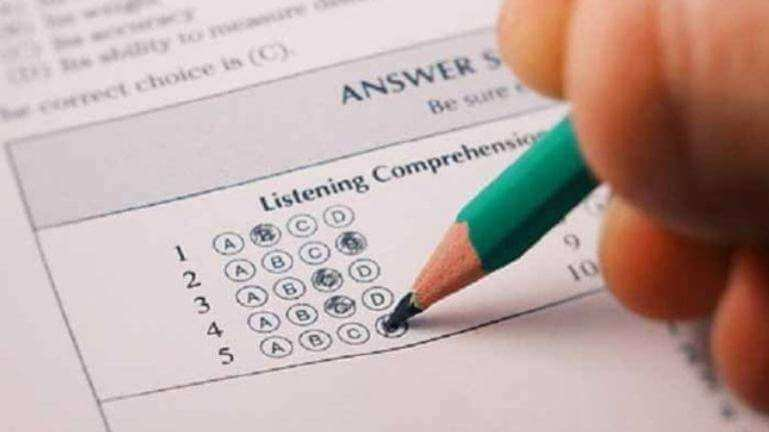# JEE MAINS 2020 MATHS weightage: Chapters you can’t skip And Preparation tips

Share with friends

Mathematics is the most vital parts of the entrance in fact, an indispensible part of human race. Chemistry, Physics and Mathematics have an equal weightage in the JEE but Mathematics has an edge over the other two as in case of a tie in the overall score of two or more candidates, the score in mathematics is considered to be the deciding factor. The subject is like the king of science which lays the foundation for Physics and Chemistry. Topics like calculus, differential equations and quadratic equations are used in almost all the questions of physics and chemistry.

IIT JEE SYLLABUS is a perfect blend of easy and difficult topics. Some topics like probability, trigonometry, differential calculus, circles and straight lines in coordinate geometry, permutations and combinations in algebra are always easy to crack iit jee.  Those days are gone when students used to spend hours in attempting one question. This is an era of multiple choice questions. Mathematics questions in the JEE test a student’s acquired knowledge as well as his aptitude.

Math JEE Main Books to Prepare well

It is advisable to not spend time reading too many books because that may leave you with no time to revise at the end. You should keep a few important books with the consultation of your teachers. Below mentioned are a few books you can refer to for cracking IIT JEE 2018 Mathematics paper:

• R.D. Sharma for Class XI & XII
• Trigonometry by S.L. Loney
• Coordinate Geometry by S.L. Loney
• Higher Algebra by Hall Knight
• Problems in Calculus of One Variable by L.A. Maron

How to prepare for JEE Mains Mathematics?

If you are wondering what to do and what not to do in Mathematics in order to crack IIT JEE in flying colors, you need to follow the tips for JEE Main Maths. If the tips are followed without any failure, you will crack the entrance in one go.

• Emphasize on Calculus and Algebra: You should be thorough with the topics under calculus and algebra. These are two of the most important topics and you need to be thorough with these two topics. Practice every day and clear the doubts as and when you come across any.
• Study all formulae: Knowing formulae in Mathematics can be a big plus point for any Math exam.  Therefore, it is all the more important that you understand all the formulas in probability, geometry and trigonometry. Again, knowing the important formulas in calculus will help you enormously.
• Be sure of the applications: You should revise the applications of differential equations and geometry. This applies for properties of definite integrals and results of algebraic calculations of properties of conics. Thorough revision of these applications should be done before you attempt IIT JEE.
• Prepare a list of formulae:  Formulae are very important in mathematics. So if you think you might have trouble remembering all of them, you can create a table including the topics and formulas from all the chapters. You should go through it every day. Over a period of time, you will be able to remember all the formulae. Ideally, morning is the best time for revising the formulae.
• Solve model papers every day: Solving two to three model questions papers will help you a lot as it will help you manage your time better and get your rhythm going. But, don’t overburden yourself as you have two other subjects as well. You should give adequate time to all.
• Start revising: When you have at least two to three months for the entrance, you should have completed everything in the syllabus and start revising. This will ensure that you have enough time to revise and get more confident with the subject. It is one of the most scoring subjects of the three. Practicing every day without a default will give you the edge.
• Previous Question Papers: When you are done with the revision too, start devoting time for solving the question papers asked in the previous years. Whenever you study tips for JEE Main Maths, you will know that solving past question papers is an important tip that you can’t ignore. This way you also get an overview of what the JEE Main Math questions are likely to be. You won’t feel strange when you attempt for the entrance for the first time. You will have a sense of already attempted and this boosts your morale too.
• Reference Materials: It is also important that you refer to the books mentioned above after the completion of NCERT books. This will give you more information and explanation about a single topic. JEE Main Maths preparation solely depends on the extensiveness of the study and reference materials help in a huge way.

Maths chapterwise weightage FOR JEE MAINS –https://drive.google.com/open?id=1vP07npD7hlQorQEq-SA83KBPnT9slrNK

Share with friends